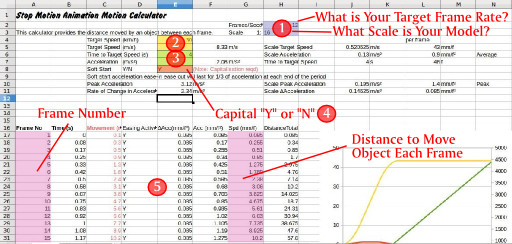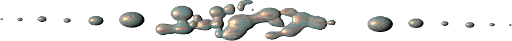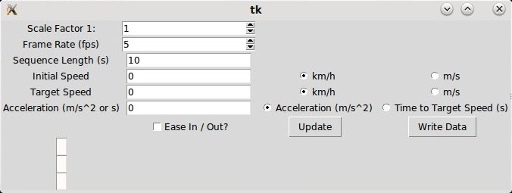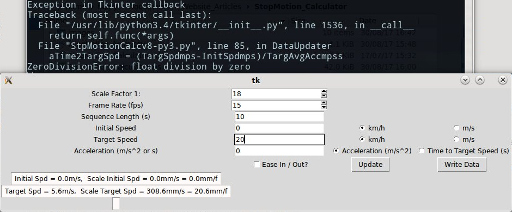## A Stop Motion Calculator

### Le Thu 31 August 2017

One of the things I quickly noticed when making the small test animation with the Minetrolley and Mine Truck, aside from the sand “Wake” caused by my cuffs, was the choppiness of the animation. This was because there was no motion blur. It got me to thinking. Two things occurred to me;

• I had no idea what speed I was simulating with the movements between each frame.
• If I were to build a “go-motion' rig, it would need some sort of calculation to work out how far to move during each frame to create some motion blur. The Camera slide project would lend itself well to this.

A calculator was in order.

I'm guessing that any experienced animator has a natural feel for what motion is required to give the appropriate illusion of speed, but for me a tool like this will help me achieve a slightly better flow while also producing an output that I can use in a motion control rig.

I made two. One is a simple spreadsheet which produces a table of results which can be used to determine the motion between successive frames. This can either be used directly, plotted, or the useful data pulled out into another file for feeding into a motion control rig. The other is a python program complete with GUI which will output a .csv file with your calculated object motion data.

# The Stopmotion Calculator Spreadsheet

The calculations are simple and should work just fine in the Excel version, but the chart might be lost. Sorry I have no way of checking.

Just to explain how to use it;Item 1. You will have in mind roughly how big your model is and what frame rate you intend to play back your animation at, so you can put those bits of information into the item identified as “1” on the diagram above.

Item 2. You will have a target speed in mind. Say you had a model car and you wanted it to represent travelling at 80km/h, you would just add 80km/h into the top box at Item “2”. Alternatively you could input the speed in as 22.2m/s but put that into the lower cell.

Item 3. There are two choices for controlling the acceleration. You can either provide an acceleration in m/s² or as a time to reach the target speed (in seconds).

Item 4. To produce a more realistic motion, there is provision for a “Soft Start” or “Ease in / Ease out”. This is a smoothing of the acceleration created by introducing a constant change in acceleration over time which acts over the first third of the time the object is accelerating, and the last third as it approaches the target speed. To is calculated automatically. All you need to do is put a capital “Y” or “N” in the cell identified by Item 4 in the diagram above.

Item 5. Here is where the chart of movements is produced. The most important columns are the Frame number (column A) and the movement between each frame (Column G) listed as mm/frame. Column H may be useful if you look at the cell in that column that represented your last frame. This is the total distance moved by the animated object over the course of your animation sequence. You will be able to determine just how big your stage needs to be.

A word of caution

The calculator is not designed to calculate the simple case of constant speed. You will get division by zero errors. You can calculate that out by hand or put in something like a very high acceleration or a very brief time to target speed. Having said that the data to the right of the data entry areas will be reporting the speed you need to move the model per frame. It's just the produced table will be a bit of a mess.# The Python Stopmotion Calculator

So, having created the spreadsheet calculator, I was suddenly taken with the irrational desire to make a python based calculator with a GUI. I had not played with tkinter or and felt that if I really wanted to make useful tools, I should get to grips with it. This seemed like a perfect opportunity.

As it happened, I extended the functionality in the script by making it able to handle initial speeds as well. Like the spreadsheet, this calculator does not calculate the trivial case where there is no acceleration at any point over the entire animation sequence.

I have created versions for Python 2.7X and Python 3.X. you can download them below:

It goes without saying you will need the Tkinter library installed if it is not already present.

Anyway, when the script is running you will be initially faced with something that looks like this;Click on the Image for a Larger View

Yeah, I know its a bit ugly, but I haven't bothered to pretty it up with some proper layout OK?

Hopefully it is all pretty self explanatory. You use the radio buttons on the right to set the units or calculation method for the acceleration. Once you have set your scale factor, frame rates and various speeds and accelerations (or time to target speed) hit the “Update” Button.

Updating goes through the calculations but does not produce an output table of measurements. The updated screen will look like this;Click on the Image for a Larger View

The message boxes in the bottom left are the calculated values for the speeds and accelerations at full scale and at your chosen scale. It also calculates the movement per frame for these key speeds and accelerations. These are expressed as mm/frame and mm/frame² just to introduce some nice dirty units.

Had I wanted a deceleration, then I can just as easily use negative accelerations and target speeds lower than the initial speed. The calculations include some safeguards to ensure acceleration values that are the wrong sign for achieving the target speed from the initial speed are ignored.

Once you are happy with your settings, hit the “Write Data” button and this will open a dialogue to create a file somewhere of your choosing. The default extension is a .csv.

If you are only wanting to use this calculator for constant motion, then the initial speed or target speed message boxes will calculate the movement per frame you require, but in the background there will be errors popping up complaining about divisions by zero etc. For instance in the trivial example below with a desired 20km/h speed, a scale of 1:18, and a 15 frame/s playback the calculator has correctly given us the scale speed as 20.6mm/frame.Click on the Image for a Larger View

## A Test Animation

For the sake of testing the output, I quickly created this little animation. I think the motion of the Mining Trolley looks better and appears to have a reasonably smooth acceleration. It was designed to accelerate to about 20km/h within 5 seconds had it been full size. the scale I used was 1:18 and the frame rate 15 frames per second. Despite rolling my sleeves up I see I was still bumping other object too much. I swear those little bricks are alive!

## Program Listing

The listing below is for the Python 3 version. Apart from some extremely minor changes, the Python 2.7 version is identical.

Python 3 version can be downloaded from here: StpMotionCalcv8-py3.py Python 2.7X version can be downloaded from here: StpMotionCalcp27xv1.py

The Python 3 version.

```# File: StpMotionCalcv8-py3.py
# This calculator uses an update button to capture all of the data provided and
# produce the interim values. The "Write Data" button outputs the data as a CSV
# file which can be plotted or potentially used in a motion rig.

# The Ease in and Ease out is a soft start calculation using the change in
# acceleration with time.  The softness is preset in code as 1/3rd of the time
# to target speed when accelerating and for the time between 2/3rds and time to
# full speed for the acceleration to be eased off.  This produces a higher
# peak acceleration.

# There is some deviation from the calculated and displayed target speeds
# when the data is charted.  This appears to be related to the fact that frames
# are not a continuous thing and so the target speeds and accelerations
# will not be exact.

# Python 3 version

import os
from tkinter import *
from tkinter import filedialog
from tkinter import messagebox

root = Tk()

def DataUpdater():
#This function goes through the available data and updates it with the most recent values.
aScaleFactDat = ScaleFact.get()
aFrameRateDat = FrameRate.get()
SeqTime = float(SeqLntxt.get())
aNoFrames = int(SeqTime * aFrameRateDat)

#Initial Speed Consolidating the Units
if InitSpdUnits.get() == 1:
#Selected unit for speed is m/s
InitSpdmps = float(InitSpdtxt.get()) #capture the text box content.

else:
#Selected unit for speed is km/h
InitSpdmps = float(InitSpdtxt.get())/3.6 #capture the text box content (km/h) and convert to m/s

InitSpdScalemmps = InitSpdmps * 1000 / aScaleFactDat
aInitSpdScalemmff = InitSpdScalemmps / aFrameRateDat
InitSpdDisplaytxt = "Initial Spd = {:.1f}m/s,  Scale Initial Spd = {:.1f}mm/s = {:.1f}mm/f".format(InitSpdmps,InitSpdScalemmps,aInitSpdScalemmff)
InitSpdLabel.config(text = InitSpdDisplaytxt)

#Target Speed Consolidating the Units
if TargetSpdUnits.get() == 1:
#Selected unit for speed is m/s
TargSpdmps = float(TargetSpdtxt.get()) #capture the text box content.

else:
#Selected unit for speed is km/h
TargSpdmps = float(TargetSpdtxt.get())/3.6 #capture the text box content (km/h) and convert to m/s

TargSpdScalemmps = TargSpdmps * 1000 / aScaleFactDat
aTargSpdScalemmff = TargSpdScalemmps / aFrameRateDat
TargSpdDisplaytxt = "Target Spd = {:.1f}m/s,  Scale Target Spd = {:.1f}mm/s = {:.1f}mm/f".format(TargSpdmps,TargSpdScalemmps,aTargSpdScalemmff)
TargSpdLabel.config(text = TargSpdDisplaytxt)

#Target Average Acceleration or Time to Target Speed Consolidating the Units
if TargetAccUnits.get() == 1:
#selected entry is time to target speed.
TargAvgAccmpss = (TargSpdmps-InitSpdmps)/float(TargetAcctxt.get())
aTime2TargSpd = float(TargetAcctxt.get())

else:
#Selected entry is an average acceleration expressed in mm/s^2
TargAvgAccmpss = float(TargetAcctxt.get())
if TargAvgAccmpss > 0 and (TargSpdmps-InitSpdmps)<0:
TargAvgAccmpss = -TargAvgAccmpss #Swap signs to correct acceleration
elif TargAvgAccmpss < 0 and (TargSpdmps-InitSpdmps)>0:
TargAvgAccmpss = -TargAvgAccmpss #Swap signs to correct acceleration

aTime2TargSpd = (TargSpdmps-InitSpdmps)/TargAvgAccmpss

TargAccScalemmpss = TargAvgAccmpss * 1000 / aScaleFactDat
aTargAccScalemmpff = TargAccScalemmpss / aFrameRateDat**2
TargAccDisplaytxt = "Avg Acc = {:.2f}m/s^2, Scale Avg Acc = {:.2f}mm/s^2 = {:.2f}mm/f^2".format(TargAvgAccmpss,TargAccScalemmpss,aTargAccScalemmpff)
AvgAccLabel.config(text = TargAccDisplaytxt)

aEaseAcc = EaseInOut.get()
if aEaseAcc == 0:
#No Easing
Accelmmpss = TargAccScalemmpss  #All calculations will use the average acceleration.
aAccelmmpff = aTargAccScalemmpff
aAccelDeltammpfff = 0
aAccelPeakmmpff = 0

else:
# Soft start and ease out are used - Note these are scaled
AccelDeltammpsss = 9/2*TargAccScalemmpss/aTime2TargSpd

aAccelDeltammpfff = AccelDeltammpsss/aFrameRateDat**3
AccelPeakmmpss = 3/2*TargAccScalemmpss
aAccelPeakmmpff = AccelPeakmmpss/aFrameRateDat**2
aAccelmmpff = aTargAccScalemmpff

# Create a list to hold all the data so it can be returned to the
# Other parts of the script.
DataBundle = [aScaleFactDat,aFrameRateDat,aNoFrames,aEaseAcc,aTime2TargSpd,aInitSpdScalemmff,aTargSpdScalemmff,aTargAccScalemmpff,aAccelmmpff,aAccelPeakmmpff,aAccelDeltammpfff]

return DataBundle

def DataWriter():
#First call the Update Function to capture the necessary data.
DataCap = DataUpdater()  #DataCap is the carrier for the information returned
# from the function.
#Unpack it all into the variables for the calculations.
ScaleFactDat = DataCap
FrameRateDat = DataCap
NoFrames = DataCap
EaseAcc = DataCap
Time2TargSpd = DataCap
InitSpdScalemmff = DataCap
TargSpdScalemmff = DataCap
TargAccScalemmpff = DataCap
Accelmmpff = DataCap
AccelPeakmmpff = DataCap
AccelDeltammpfff = DataCap

# Get the filename and location to output to using a SaveAs dialogue box.
fileoutname = filedialog.asksaveasfilename(title="Please select a file name for saving:", defaultextension=".csv")
if fileoutname is None: # asksaveasfile return `None` if dialog closed with "cancel".
return

FileOut = open(fileoutname,'w')
OutputStrHeadings = "Frame No,Time(s),DeltaAcc(mm/f^3),Accel(mm/f^2),Speed(mm/f),Total Dist(mm)\n"

#Create the list of frame numbers.
FrameNoList = list(range(1,NoFrames+1)) #List of frame numbers
ListSize = len(FrameNoList)  # This gives the size of the list to use in future.
TimesList = *ListSize #Create an empty list to use.
for ff in range(0,NoFrames):
TimesList[ff] = ff/FrameRateDat  #List of times in seconds for each frame.
DeltaAccList = *ListSize  #This fills the DeltaAccList with zeros

#Calculate the deltaAcceleration list - scaled values based on frame rates.
if EaseAcc == 1:
# Easing is used.  In this case the first 1/3 of the time to target speed
# is at AccelDeltammpsss and the last 1/3 approaching the target speed
# is at a -AccelDeltammpsss.  If Easing is not used, the zero filled
# DeltaAccList is good.  Note:  these are scaled values already.
for t in range(0,NoFrames):
if TimesList[t] < Time2TargSpd/3:
DeltaAccList[t] = AccelDeltammpfff
elif TimesList[t] > Time2TargSpd/3 and TimesList[t] < 2*Time2TargSpd/3:
DeltaAccList[t] = 0
elif TimesList[t] > 2*Time2TargSpd/3 and TimesList[t] <= Time2TargSpd:
DeltaAccList[t] = -AccelDeltammpfff
else:
DeltaAccList[t] = 0

#Calculate the Acceleration list
AccScaledList = *ListSize  #Initialise the list.
if EaseAcc == 0:
for t in range(0,NoFrames):
if TimesList[t] < Time2TargSpd:
AccScaledList[t]=Accelmmpff #Average scaled acceleration
else:
AccScaledList[t]=0

else:
AccHoldermmpff = 0  #This we use to accumulate the accelerations
for t in range(0,NoFrames):
AccHoldermmpff = AccHoldermmpff + DeltaAccList[t] #Frame by frame
# basis accumulation of DeltaAccelerations
AccScaledList[t] = AccHoldermmpff
if TimesList[t] > Time2TargSpd:
AccScaledList[t] = 0

#Calculate the Speed list (as this is a distance per frame) this is the
#actual output we are looking for.

SpdScaledlist = *ListSize  #Initialise the list.
SpdHoldermmpf = InitSpdScalemmff # Accumulator for the speeds but with initial speed
# added as the starting point (naturally)
for t in range(0,NoFrames):
SpdHoldermmpf = SpdHoldermmpf + AccScaledList[t]
SpdScaledlist[t]=SpdHoldermmpf

#Calculate the Total Distance List

TotDistlist = *ListSize  #Initialise the list.
DistHoldermm = 0 #Accumulator for the distances
for t in range(0,NoFrames):
DistHoldermm = DistHoldermm + SpdScaledlist[t]
TotDistlist[t] = DistHoldermm

#Output these figures to the file as a CSV.
#Construct the string.
for t in range(0,NoFrames):
OutputStrLine = str(t+1)+",{:.2f},{:.2f},{:.2f},{:.1f},{:.0f}\n".format(TimesList[t],DeltaAccList[t],AccScaledList[t],SpdScaledlist[t],TotDistlist[t])
FileOut.writelines(OutputStrLine)

FileOut.close()
messagebox.showinfo(title = "File Created", message = "Tah!  Your file has now been created")
print("Done - File Written")

#Somewhere to enter the scale factor
ScaleLabel = Label(root,text="Scale Factor 1:")
ScaleLabel.grid(row=0,column=0)

ScaleFact = IntVar()
SclFactEntryBox = Spinbox(root,textvariable = ScaleFact,from_=1, to=50)
SclFactEntryBox.grid(row=0,column=1)

#Entry for the frame rate
FrameRtLabel = Label(root,text="Frame Rate (fps)")
FrameRtLabel.grid(row=1,column=0)

FrameRate = IntVar()
FRateEntryBox = Spinbox(root,textvariable = FrameRate,from_=5,to=30)
FRateEntryBox.grid(row=1,column=1)

#Entry for length of sequence
SeqLengthLabel = Label(root,text="Sequence Length (s)")
SeqLengthLabel.grid(row=2,column=0)

SeqLntxt = StringVar()
SeqLntxt.set("10")
SeqLnEntryBox = Entry(root,textvariable=SeqLntxt)
SeqLnEntryBox.grid(row=2,column=1)

#Entry for the Inital Speed
#For this we'll use a pair of radio type selectors to indicate the units used.

InitSpdLabel = Label(root,text="Initial Speed")
InitSpdLabel.grid(row=3,column=0)

InitSpdtxt = StringVar()
InitSpdtxt.set("0")
InitSpdEntryBox=Entry(root,textvariable=InitSpdtxt)
InitSpdEntryBox.grid(row=3,column=1)

InitSpdUnits = IntVar()
InitSpdUkmh = Radiobutton(root,text = "km/h",variable = InitSpdUnits,value=0)
InitSpdUkmh.grid(row=3,column=2)
InitSpdUmps = Radiobutton(root,text = "m/s",variable = InitSpdUnits,value=1)
InitSpdUmps.grid(row=3,column=3)

#Entry for the Target Speed
#For this we'll use a pair of radio type selectors to indicate the units used.

TargetSpdLabel = Label(root,text="Target Speed")
TargetSpdLabel.grid(row=4,column=0)

TargetSpdtxt = StringVar()
TargetSpdtxt.set("0")
TargetSpdEntryBox=Entry(root,textvariable=TargetSpdtxt)
TargetSpdEntryBox.grid(row=4,column=1)

TargetSpdUnits = IntVar()
TargetSpdUkmh = Radiobutton(root,text = "km/h",variable = TargetSpdUnits,value=0)
TargetSpdUkmh.grid(row=4,column=2)
TargetSpdUmps = Radiobutton(root,text = "m/s",variable = TargetSpdUnits,value=1)
TargetSpdUmps.grid(row=4,column=3)

#Entry for the Time to Target Speed or Average Acceleration

TargetAccLabel = Label(root,text="Acceleration (m/s^2 or s)")
TargetAccLabel.grid(row=5,column=0)

TargetAcctxt = StringVar()
TargetAcctxt.set("0")
TargetAccEntryBox=Entry(root,textvariable=TargetAcctxt)
#TargetAccEntryBox.bind('<Return>', TargAccUnitSel)     #This binds the Enter key for entering data.
TargetAccEntryBox.grid(row=5,column=1)

TargetAccUnits = IntVar()
TargetAccUmpss = Radiobutton(root,text = "Acceleration (m/s^2)",variable = TargetAccUnits,value=0)
TargetAccUmpss.grid(row=5,column=2)
TargetAccUTTS = Radiobutton(root,text = "Time to Target Speed (s)",variable = TargetAccUnits,value=1)
TargetAccUTTS.grid(row=5,column=3)

EaseInOut = IntVar()  #set the variable to accept the state of the checkbox.

EaseInOutCk = Checkbutton(root,text="Ease In / Out?",variable = EaseInOut)
EaseInOutCk.grid(row=6,column=1)

UpdateButton = Button(root,text="Update", command = DataUpdater)
UpdateButton.grid(row=6,column=2)

CalcButton = Button(root,text="Write Data", command = DataWriter)
CalcButton.grid(row=6,column=3)

InitSpdLabel = Message(root,width = 512,bg='snow', relief=SUNKEN)
InitSpdLabel.grid(row=7,column=0)
TargSpdLabel = Message(root,width = 512,bg='snow', relief=SUNKEN)
TargSpdLabel.grid(row=8,column=0)
AvgAccLabel = Message(root,width = 512,bg='snow', relief=SUNKEN)
AvgAccLabel.grid(row=9,column=0)

root.mainloop()
```